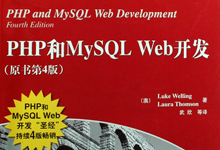# 第一章：PHP快速入门（三）

1.8 声明和使用常量

[php]
//使用define函数定义常量：
define(‘TIREPRICE’, 100);
define(‘OILPRICE’, 10);
define(‘SPARKPRICE’, 4);
[/php]

[php]echo TIREPRICE;[/php]

1.9 理解变量的作用域

PHP具有6项基本的作用域规则：
1）内置超级全局变量可以在脚本的任何地方使用和可见；
2）常量，一旦被声明，将可以在全局可见；也就是说，他们可以在函数内外使用；
3）在一个脚本中声明的全局变量在整个脚本中是可见的，但不是在函数内部；
4）函数内部使用的变量声明为全局变量时，其名称要与全局变量名称一致；
5）在函数内部创建并被声明为静态的变量无法在函数外部可见，但是可以在函数的多次执行过程中保持该值；
6）在函数内部创建的变量对函数来说是本地的，而当函数终止时，该变量也就不存在了；

1）\$GLOBAL，所有全局变量数据；
2）\$_SERVER，服务器环境变量数组；
3）\$_GET，通过GET方法传递给该脚本的变量数组；
4）\$_POST，通过POST方法传递给该脚本的变量数组；
5）\$_COOKIE，cookie变量数组；
6）\$_FILES，与文件上传相关的变量数组；
7）\$_ENV，环境变量数组；
8）\$_REQUEST，所有用户输入的变量数组，包括\$_GET、\$_POST和\$_COOKIE所包含的输入内容；
9）\$_SESSION，会话变量数组；
1.10 使用操作符

1.10.1 算术操作符

PHP中的算术操作符

 + 加 \$a+\$b – 减 \$a-\$b * 乘 \$a*\$b / 除 \$a/\$b % 取余 \$a%\$b

1.10.2 字符串操作符

1.10.3 赋值操作符

[php]\$totalqty = 0; //这句应该读成"\$totalqty"被设置为0[/php]
1）赋值运算返回值
\$a+\$b 这个表达式的值就是将\$a+\$b加在一起所得到的结果。

2）复合赋值操作符
\$a += 5 等价于 \$a = \$a+5

PHP中的复合赋值操作符

+= \$a += \$b \$a = \$a+\$b
-= \$a -= \$b \$a = \$a-\$b
*= \$a *= \$b \$a = \$a*\$b
/= \$a /= \$b \$a = \$a/\$b
%= \$a %= \$b \$a = \$a%\$b
.= \$a .= \$b \$a = \$a.\$b

3）前置递增递减和后置递增递减运算符

[php]
\$a = 4;
echo ++\$a;
[/php]

[php]
\$a = 4;
echo \$a++;
[/php]

4）引用操作符

[php]
\$a = 5;
\$b = \$a;
[/php]

[php]\$a = 7; //\$b的值还是5[/php]

[php]
\$a = 5;
\$b = &\$a;
\$a = 7; //\$a与\$b的值都为7
[/php]

[php]unset(\$a);[/php]